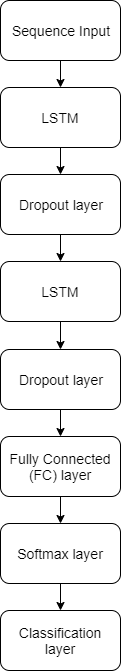## Support for Long Short-Term Memory Networks

A long short-term memory (LSTM) network is a type of recurrent neural network (RNN) that can learn long-term dependencies between time steps of sequence data. Deep Learning HDL Toolbox™ supports these LSTM network architectures:

• Single LSTM layer networks — A single LSTM layer network consists of only one LSTM layer. This diagram illustrates the architecture of a single LSTM layer network for sequence regression. The network starts with a sequence input layer followed by an LSTM layer. The network ends with a fully connected layer and a regression output layer.• Stacked LSTM layer networks — A stacked LSTM layer network consists of multiple LSTM layers. In a stacked LSTM layer network, the preceding LSTM layer provides a sequence output to the following LSTM layer. This diagram illustrates the architecture of a stacked LSTM layer network used for classification. The network starts with a sequence input layer followed by an LSTM layer, dropout layer, second LSTM layer, and a second dropout layer. To predict class labels, the network ends with a fully connected layer, a softmax layer, and a classification output layer.Deep Learning HDL Toolbox does not support bidirectional LSTM layers. For a list of supported layers, see Supported Layers.

### Prediction and Forecasting

To make predictions on new data in an LSTM network, use `predict`. See Run Sequence-to-Sequence Classification on FPGAs by Using Deep Learning HDL Toolbox.

LSTM networks can remember the state of the network between predictions. The network state is useful when you do not have the complete time series in advance, or if you want to make multiple predictions on a long time series. To predict parts of a time series and update the network state, use `predictAndUpdateState`. To reset the network state between predictions, use `resetState`. To learn about forecasting future time steps of a sequence, see Run Sequence Forecasting on FPGA by Using Deep Learning HDL Toolbox.